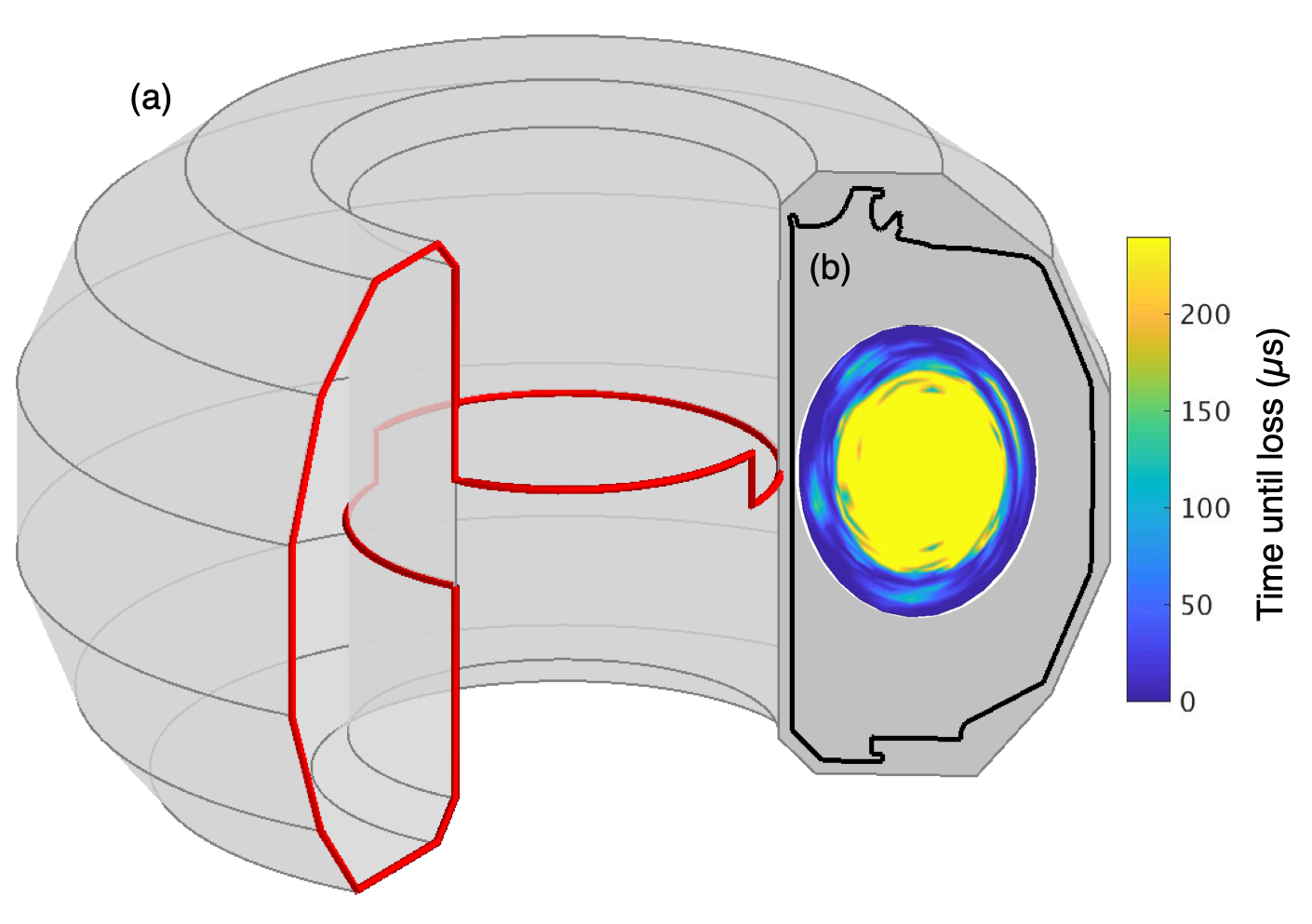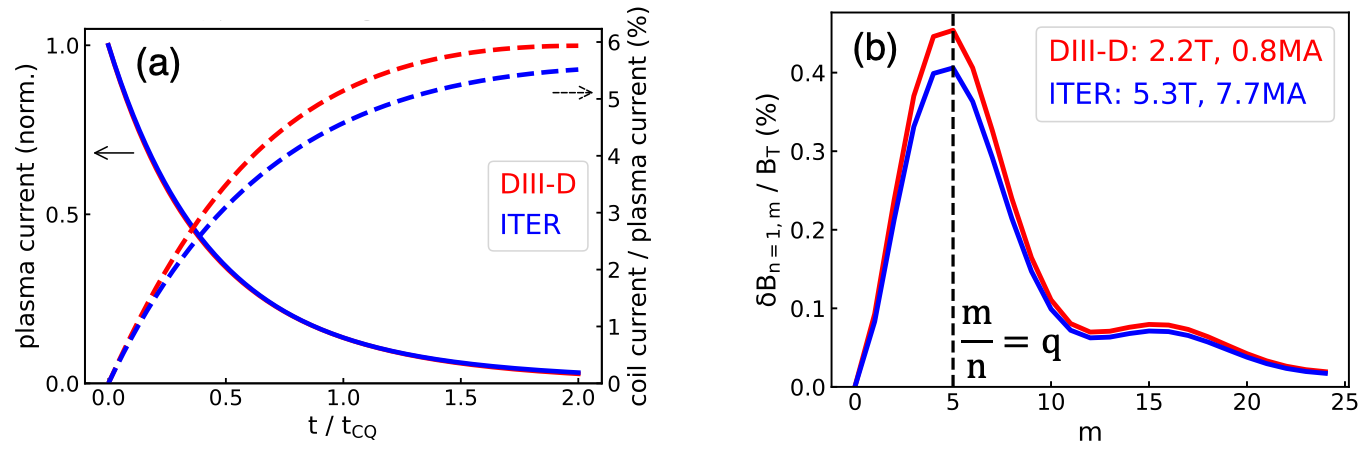Since 18 of December 2019 conferences.iaea.org uses Nucleus credentials. Visit our help pages for information on how to Register and Sign-in using Nucleus.

# 28th IAEA Fusion Energy Conference (FEC 2020)

May 10 – 15, 2021
Virtual Event
Europe/Vienna timezone
The Conference will be held virtually from 10-15 May 2021

## Passive deconfinement of runaway electrons using an in-vessel helical coil

May 12, 2021, 8:30 AM
4h
Virtual Event

#### Virtual Event

Regular Poster Magnetic Fusion Theory and Modelling

### Speaker

David Weisberg (General Atomics)

### Description

A helical coil designed to passively generate non-axisymmetric fields during a plasma disruption has been shown (via electromagnetic circuit and linear MHD modeling) to be effective at deconfining runaway electrons (REs) before the RE beam current grows to dangerous levels. Magnetic equilibria from DIII-D RE experiments were used to calculate the toroidal electric field generated during the current quench phase of a disruption, which in turn drives current in a proposed n=1, m=1 in-vessel helical coil (Fig.1), without the need for any external power supplies or disruption detection diagnostics. Modeling using both the JFIT and TokSys codes predicts coil currents up to 6% of pre-disruption plasma current (Fig.2). The coil geometry was systematically varied to maximize the magnetic perturbation at the plasma rational surfaces, resulting in an optimized coil where $\delta$B/B~$10^{-2}$. The new REORBIT module of the MARS-F code was used to model the full non-axisymmetric magnetic field and trace RE orbits to determine the coil’s effect on RE deconfinement, with up to 45% of the RE population lost after 200$\mu$s. Electromagnetic and thermal stresses on the coil are calculated to be well within operational limits for installation in DIII-D, and scale favorably to a reactor-size device.An optimized coil geometry was constructed with the goal of maximizing the non-axisymmetric magnetic perturbation in the disrupting plasma. Equilibrium fits from runaway electron experiments in DIII-D were used to approximate the magnetic geometry for field harmonic calculations. The coil geometry was parametrized in several ways, and the optimal shape was found to have shallow pitch angle and few discrete horizontal and vertical segments, localized at the midplane center-post (Fig.1a). This position minimizes the distance between coil and the disrupting plasma, maximizing the loop voltage along the coil as well as the magnetic perturbation amplitude in the plasma. This design also avoids the large number of outboard ports present on DIII-D and other tokamaks.In order to estimate the toroidal electric field generated during a disruption, the JFIT and TokSys codes were used to model the inductively coupled coil-plasma-vessel system. Both models used full 3D inductive coupling terms to calculate the evolution of the axisymmetric plasma current, predicting peak coil currents of up to 6% of the initial plasma current which are well in excess of the theoretical 2% threshold for meaningful RE deconfinement {1}. Most importantly, the coil inductance and resistance are suitable to match the disruption current quench timescale (Fig.2a) (14ms in DIII-D, 190ms in ITER). This allows the coil to passively respond to the disruption and generate a 3D magnetic perturbation with the necessary amplitude and timing for runaway electron deconfinement. The resulting vacuum field amplitude is greater than the theoretical threshold of $\delta$B/B~$10^{-3}$ {2}, and is well-matched to the edge plasma helicity (Fig. 2b).

The amount of RE deconfinement caused by this optimized coil was modeled using the new REORBIT module of the linear MHD code MARS-F {3}. After calculating the full non-axisymmetric magnetic field for toroidal mode numbers up to n=6, the REORBIT code traces test particles along field lines to determine their confinement time as a function of initial energy and pitch angle. Simulations extending out to 240$\mu$s post-disruption found that 18% of the total RE population was deconfined by expected coil currents (6% I$_\text{P}$). Doubling the coil current (12% I$_\text{P}$) further increased the loss fraction to 45% of the total RE population (Fig.1b). Unlike previous studies {4} these results do not require significant resonant plasma response or island formation; in fact, the modeled plasma response in this study was found to be negligible even though the vacuum magnetic perturbation is well-aligned with the edge helicity. Thus it is likely that these results are pessimistic, and that equilibria with lower q$_{\text{edge}}$ would show increased RE deconfinement due to non-trivial resonant response.

Scaling estimates are favorable for the operation of a passive RE deconfinement coil on a larger reactor-scale device, with only marginal decreases in relative coil current and magnetic perturbation amplitude (Fig.2). The DIII-D coil-plasma-vessel system was scaled up by a factor of 3.75 to approximate an ITER-sized device while maintaining the same edge safety factor and aspect ratio. Interestingly, these constraints dictate that I$_\text{coil}$/I$_\text{P}$ and $\delta$B/B are independent of size; the slight differences in Fig.2 are due solely to the change in vacuum vessel conductivity (Inconel to stainless steel). The mechanical and thermal stresses of such a coil on an ITER-sized device are only slightly larger than the DIII-D case. Even considering the worst-case high-current scenarios in scaling from the DIII-D (I$_\text{P}$ = 2MA) to ITER (I$_\text{P}$ = 15MA) sizes, J$\times$B stress on the coil increases from 30% to 40% of stainless steel tensile yield strength and total coil temperature rise due to joule heating increases from $\Delta$T = 20$^{\circ}$C to 50$^{\circ}$C.

In summary, a helical in-vessel coil was designed with the goal of passively deconfining REs prior to energetic beam formation via large non-axisymmetric magnetic perturbations. The linear MHD code MARS-F was used to test this hypothesis, and the coil was found to be effective at substantially increasing RE orbit losses in DIII-D equilibria. Such a coil is robust to mechanical and thermal stresses, reacts passively and quickly to a disruption event, and scales favorably to larger reactor-size devices. The experimental confirmation of these findings on an existing tokamak should be a high-priority goal of the international fusion community, and serious thought should be given to its applicability to future fusion devices.

Work supported by General Atomics Internal funds.
{1} Boozer, Plasma. Phys. Control Fusion 53 (2011) 084022
{2} Boozer, Phys. Plasmas 19 (2012) 058101
{3} Liu, Parks, Paz-Soldan, Kim, and Lao, Nucl. Fusion 59 (2019) 126021
{4} Smith, Boozer, and Helander, Phys. Plasmas 20 (2013) 072505

Affiliation General Atomics United States

### Primary author

David Weisberg (General Atomics)

### Co-authors

Carlos Paz-Soldan (General Atomics) Yueqiang Liu (General Atomics, PO Box 85608, San Diego, CA 92186-5608, USA) Brendan Lyons (General Atomics) Mr Anders Welander (General Atomics)

### Presentation materials

 TH:P3-20_weisberg.mp4 weisberg_poster_final.pdf